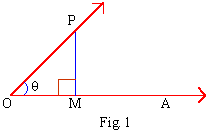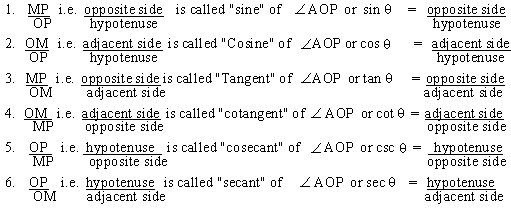Support the Monkey! Tell All your Friends and TeachersHome MonkeyNotes Printable Notes Digital Library Study Guides Study Smart Parents Tips College Planning Test Prep Fun Zone Help / FAQ How to Cite New Title Request

CHAPTER 2 : TRIGONOMETRIC FUNCTIONS OF ACUTE ANGLES

2.1 Trigonometric Ratios of Acute Angles

The building blocks of trigonometry is the characteristics of similar triangles, originally formulated by 'Euclid'.

If two corresponding angles are congruent then the two triangles are similar. Also corresponding sides of two similar triangles preserve the same ratio. This idea leads to the trigonometric ratios. For trigonometric ratios of acute angles, we need right angled triangles. The measure of an acute angle in a right angled triangle, lower case Greek letters a and q are usually used.Let a revolving line OP starts from OA and revolve into a position OP, thus tracing out the angle AOP. Draw seg PM, perpendicular to the initial line OA. Let Ð POM = q.

In the right angled triangle POM, OP is the hypotenuse, PM is the opposite side of q and OM is the adjacent side of q. Now trigonometric ratios, or functions are relation between any two sides of a right angle triangle with respect to a given angle. Thus a trigonometric ratio is a function of an angle.Your browser does not support the IFRAME tag.

The trigonometric ratios of Ð POM = q are defined as follows :It should be noted that the trigonometric ratios are all numbers

IndexSearch: All Products Books Popular Music Classical Music Video DVD Toys & Games Electronics Software Tools & Hardware Outdoor Living Kitchen & Housewares Camera & Photo Cell Phones Keywords: